Courses

# Test: Work, Energy And Power 1 - From Past 28 Years Questions

## 23 Questions MCQ Test Physics 28 Years Past year papers for NEET/AIPMT Class 11 | Test: Work, Energy And Power 1 - From Past 28 Years Questions

Description
This mock test of Test: Work, Energy And Power 1 - From Past 28 Years Questions for NEET helps you for every NEET entrance exam. This contains 23 Multiple Choice Questions for NEET Test: Work, Energy And Power 1 - From Past 28 Years Questions (mcq) to study with solutions a complete question bank. The solved questions answers in this Test: Work, Energy And Power 1 - From Past 28 Years Questions quiz give you a good mix of easy questions and tough questions. NEET students definitely take this Test: Work, Energy And Power 1 - From Past 28 Years Questions exercise for a better result in the exam. You can find other Test: Work, Energy And Power 1 - From Past 28 Years Questions extra questions, long questions & short questions for NEET on EduRev as well by searching above.
QUESTION: 1

### A 0.5 kg ball moving with speed of 12 m/s strikes a hard wall at an angle of 30° with the wall. It is reflected with the same speed and at the same angle. If the ball is in contact with the wall for 0.25 seconds, the average force acting on the wall is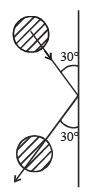Solution: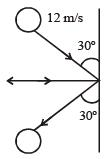Resolving the velocities in vertical and horizontal directions, resolved parts of first velocity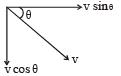v cosθ perpendicular to the wall and v sinθ parallel to the wall. In the second case, they are –v sinθ & v cosθ respectively. Here, –ve sign is because direction is opposite to the earlier ones. So we see a net change in velocity perpendicular to way = v sinθ – (–v sinθ) = 2v sinθ
This change has occured in 0.25 sec, so, rate  of change of velocity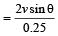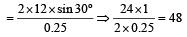Thus, acceleration a = 48 m/sec2

Force applied = m . a = 0.5 × 48 = 24 N

QUESTION: 2

### A vertical spring with force constant k is fixed on a table. A ball of mass m at a height h above the free upper end of the spring falls vertically on the spring so that the spring is compressed by a distance d. The net work done in the process is  

Solution:

Gravitational potential energy of ball gets converted into elastic potential energy of the spring.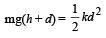Net work done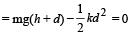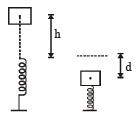QUESTION: 3

### Water falls from a height of 60 m at the rate of 15 kg/s to operate a turbine. The losses due to frictional force are 10% of energy. How much power is generated by the turbine?( g = 10 m/s2)

Solution:

Given, h = 60m, g = 10 ms–2, Rate of flow of water = 15 kg/s

∴ Power of the falling water = 15 kgs–1 × 10 ms–2 × 60 m = 900 watt.
Loss in energy due to friction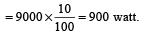∴ Power generated by the turbine = ( 9000 – 900) watt = 8100 watt = 8.1 kW

QUESTION: 4

A shell of mass 200 gm is ejected from a gun of mass 4 kg by an explosion that generates 1.05 kJ of energy. The initial velocity of the shell is:

Solution:

Let the initial velocity of the shell be v, then by the conservation of momentum mv = Mv' where v' = velocity of gun.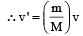Now, total K.E.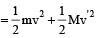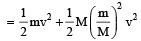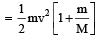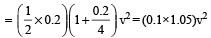But total K.E. = 1.05 kJ = 1.05 × 103J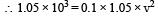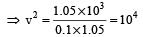∴ v = 102 = 100 ms–1.

QUESTION: 5

An engine pumps water continuously through a hose. Water leaves the hose with a velocity v and m is the mass per unit length of the water jet. What is the rate at which kinetic energy is imparted to water? 

Solution:

m : mass per unit length

∴  rate of mass leaving the the hose per sec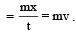Rate of K.E.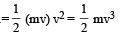QUESTION: 6

An explosion blows a rock into three parts. Two parts go off at right angles to each other. These two are, 1 kg first part moving with a velocity of 12 ms–1 and 2 kg second part moving with a velocity of 8 ms–1. If the third part flies off with a velocity of 4 ms–1, its mass would be: 

Solution:

Let two parts of the rock move along x-axis and y-axis respectively.
If M and v be the mass and velocities of third part then
Mv cosθ = 12
Mv sinθ =16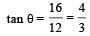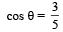v = 4 m/s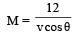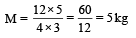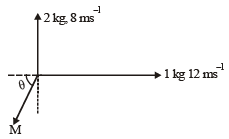QUESTION: 7

A body of mass 1 kg is thrown upwards with a velocity 20 m/s. It momentarily comes to rest after attaining a height of 18 m. How much energy is lost due to air friction? (g = 10 m/s2)

Solution:

When the body is thrown upwards. its K.E is converted  into P.E. The loss of energy due to air friction is the difference of K.E and P.E.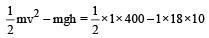= 200 – 180 = 20 J

QUESTION: 8

A ball moving with velocity 2 m/s collides head on with  another stationary ball of double the mass. If the coefficient of restitution is 0.5, then their velocities (in m/s) after  collision will be:

Solution: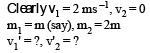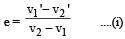By conservation of momentum, 2m = mv1' + 2mv2' ... (ii) From (i),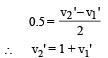From (ii), 2 = v1'+ 2 + 2 v1'

⇒  v1 = 0 and v2 = 1 ms–1

QUESTION: 9

An engine pumps water through a hose pipe.Water passes through the pipe and leaves it with a velocity of 2 m/s. The mass per unit length of water in the pipe is 100 kg/m. What is the power of the engine? 

Solution:

Solution :- Amount of water flowing per second from the pipe

= m/time

= (m/l) *(l/t)

= (m/l)

Power = KE of water flowing per second

= 1/2(m/l)v.v^2

= 1/2(m/l)v3

= 12×100×8=400W

QUESTION: 10

The potential energy of a system increases if work is done 

Solution:

The potential energy of a system increase if work is done by the system against a conservative force.
−ΔU = Wconservative force

QUESTION: 11

A body projected ver tically fr om the ear th reaches a height equal to earth's radius before returning to the earth. The power exerted by the gravitational force is greatest 

Solution:

Power exerted by a force is given by P = F.v
When the body is just above the earth’s surface, its velocity is greatest. At this instant, gravitational force is also maximum.
Hence, the power exerted by the gravitational force is greatest at the instant just before the body hits the earth.

QUESTION: 12

Force F on a particle moving in a straight line varies with distance d as shown in the figure.The work done on the particle during its displacement of 12 m is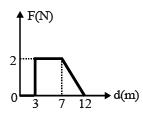Solution:

Work done = area under F-d graph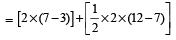= 8 + 5
= 13 J.

QUESTION: 13

A mass m moving horizontally (along the x-axis) with velocity v collides and sticks to mass of 3m moving vertically upward (along the y-axis) with velocity 2v. The final velocity of the combination is [2011M]

Solution:

As the two masses stick together after collision, hence it is inelastic collision.
Therefore, only momentum is conserved.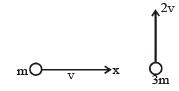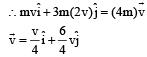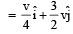QUESTION: 14

A solid cylinder of mass 3 kg is rolling on a horizontal surface with velocity 4 ms–1. It collides with a horizontal spring of force constant 200 Nm–1 . The maximum compression produced in the spring will be : 

Solution:

At maximum compression the solid cylinder will stop so loss in K.E. of cylinder = gain in P.E. of spring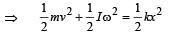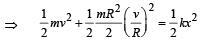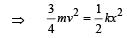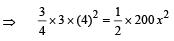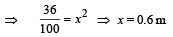QUESTION: 15

Two sph eres A and B of masses m1 and m2 respectively collide. A is at rest initially and B is moving with velocity v along x-axis. After collision B has a velocity 2v  in a directionperpendicular to the original direction. The mass A moves after collision in the direction.

Solution: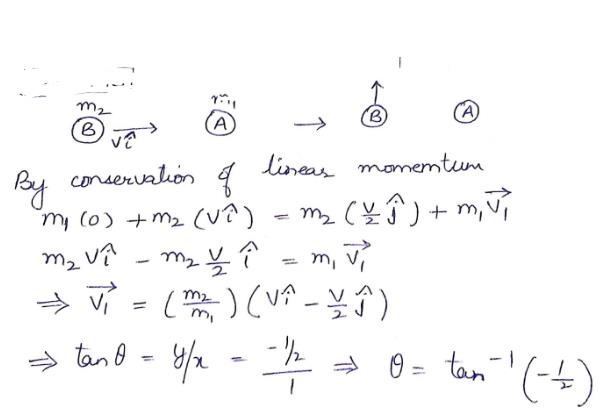Answer :- c

Solution :- Here initially sphere A is at rest and B is moving along x axis with velocity V.

After collision, velocity of sphere B becomes V/2 along direction perpendicular to its initial direction i.e. along Y axis.

Sphere A will move with some velocity u at some angle θ as shown in figure.considering law of conservation of momentum along X−axis.

m2V=m1ucosθ...........(i)

Considering law of conservation of momentum along Y−axis.

m2v/2=m1usinθ............(ii)

From equation i and ii.

1/2=tanθ

θ=tan−1(0.5).

QUESTION: 16

A car of mass m starts from rest and accelerates so that the instantaneous power delivered to the car has a constant magnitude P0. The instantaneous velocity of this car is proportional to : [2012M]

Solution:

Constant power of car P0 = F.V = ma.v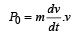P0dt = mvdv Integrating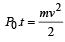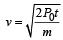∵  P0, m and 2 are constant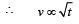QUESTION: 17

A uniform force of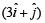newton acts on a particle of mass 2 kg. The particle is displaced from position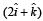meter to position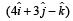meter. The work done by the force on the particle is [NEET 2013]

Solution: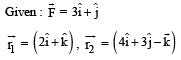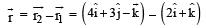So work done by the given force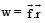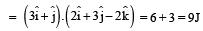QUESTION: 18

The upper half of an inclined plane of inclination θ is perfectly smooth while lower half is rough.
A block starting from rest at the top of the plane will again come to rest at the bottom, if the coefficient of friction between the block and lower half of the plane is given by [NEET 2013]

Solution: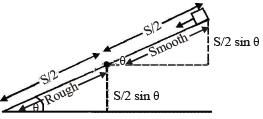For upper half of inclined  plane v2 = u2 + 2a S/2 = 2 (g sinθ) S/2 = gS sinθ
For lower half of inclined plane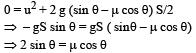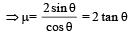QUESTION: 19

An explosion breaks a rock into three parts in a horizontal plane. Two of them go off at right angles to each other. The first part of mass 1 kg moves with a speed of 12 ms–1 and the second part of mass 2 kg moves with speed 8 ms–1. If the third part flies off with speed 4 ms–1 then its mass is [NEET 2013]

Solution: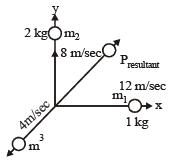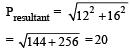m3v3 = 20 (momentum of third part)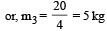QUESTION: 20

An explosion breaks a rock into three parts in a horizontal plane. Two of them go off at right angles to each other. The first part of mass 1 kg moves with a speed of 12 ms–1 and the second part of mass 2 kg moves with speed 8 ms–1. If the third part flies off with speed 4 ms–1 then its mass is [NEET 2013]

Solution: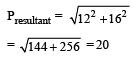m3v3 = 20 (momentum of third part)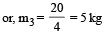QUESTION: 21

A person holding a rifle (mass of person and rifle together is 100 kg) stands on a smooth surface and fires 10 shots horizontally, in 5 s.Each bullet has a mass of 10 g with a muzzle velocity of 800 ms–1. The final velocity acquired by the person and the average force exerted on the person are [NEET Kar. 2013]

Solution:

We have to use the Law of momentum conservation:

P ( initial ) = P ( final )

0 = n · m · u + ( M - n · m ) · v

where: n = 10, m = 10 g = 0.01 kg, u = 800 m/s, M = 100 kg.

0 = 10 · 0.01 kg · 800 m/s + ( 100 kg - 10 · 0.01 kg ) · v

v = - 80 kgm/s / 99.9 kgm/s

v = 0.8 m/s

Then : F = Δ P / Δ t = ( 10 · 0.01 kg · 800 m/s ) : 5 s = 16 N

Answer: The average force exerted on the person is 16 N.

QUESTION: 22

A particle with total energy E is moving in a potential energy region U(x). Motion of the particle is restricted to the region when [NEET Kar. 2013]

Solution:

As the particle is moving in a potential energy region.

∴ Kinetic energy ≥ 0 And, total energy E = K.E. + P.E.

⇒ U(x) ≤ E

QUESTION: 23

One coolie takes 1 minute to raise a suitcase through a height of 2 m but the second coolie takes 30 s to raise the same suitcase to the same height. The powers of two coolies are in the ratio of [NEET Kar. 2013]

Solution: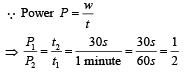(t1 = 1 minute; t2 = 30 second given)